SLOS417D October   2003  – November 2015

PRODUCTION DATA.

1. Features
2. Applications
3. Description
4. Device Comparison Table
5. Pin Configuration and Functions
6. Specifications
7. Parameter Measurement Information
8. Detailed Description
1. 8.1 Overview
2. 8.2 Functional Block Diagram
3. 8.3 Feature Description
4. 8.4 Device Functional Modes
9. Application and Implementation
1. 9.1 Application Information
2. 9.2 Typical Applications
1. 9.2.1 TPA200110D1 With Differential Input
2. 9.2.2 TPA20010D1 With Differential Input and Input Capacitors
3. 9.2.3 TPA20010D1 with Single-Ended Input
10. 10Power Supply Recommendations
11. 11Layout
1. 11.1 Layout Guidelines
2. 11.2 Board Layout
3. 11.3 Layout Example
12. 12Device and Documentation Support
13. 13Mechanical, Packaging, and Orderable Information

• YZF|9

## 8 Detailed Description

### 8.1 Overview

The TPA2010D1 is a high-efficiency filter-free Class-D audio amplifier capable of delivering up to 2.5 W into 4-Ω loads with 5-V power supply. The fully-differential design of this amplifier avoids the usage of bypass capacitors and the improved CMRR eliminates the usage of input-coupling capacitors. This makes the device size a perfect choice for small, portable applications because only three external components are required. The advanced modulation used in the TPA2010D1 PWM output stage eliminates the need for an output filter.

### 8.2 Functional Block Diagram### 8.3 Feature Description

#### 8.3.1 Fully Differential Amplifier

The TPA2010D1 is a fully differential amplifier with differential inputs and outputs. The fully differential amplifier consists of a differential amplifier and a common-mode amplifier. The differential amplifier ensures that the amplifier outputs a differential voltage on the output that is equal to the differential input times the gain. The common-mode feedback ensures that the common-mode voltage at the output is biased around VDD/2 regardless of the common-mode voltage at the input. The fully differential TPA2010D1 can still be used with a single-ended input; however, TI recommends using the TPA2010D1 with differential inputs when in a noisy environment, like a wireless handset, to ensure maximum noise rejection.

#### 8.3.2 Advantages of Fully Differential Amplifiers

• Input-coupling capacitors not required:
• The fully differential amplifier allows for input bias at voltage other than mid-supply. For example, if a codec has a midsupply lower than the midsupply of the TPA2010D1, the common-mode feedback circuit adjusts, and the TPA2010D1 outputs remain biased at midsupply of the TPA2010D1. The inputs of the TPA2010D1 can be biased from 0.5 V to VDD –0.8 V. If the inputs are biased outside of that range, input-coupling capacitors are required.
• Midsupply bypass capacitor, C(BYPASS), not required:
• The fully differential amplifier does not require a bypass capacitor because any shift in the midsupply affects both positive and negative channels equally and cancels at the differential output.
• Better RF-immunity:
• GSM handsets save power by turning on and shutting off the RF transmitter at a rate of 217 Hz. The transmitted signal is picked up on input and output traces. The fully differential amplifier cancels the signal better than the typical audio amplifier.

#### 8.3.3 Efficiency and Thermal Information

The maximum ambient temperature depends on the PCB system heatsinking ability. The derating factor for the YEF and YEZ packages appear in the dissipation rating table. Converting this to θJA:

Equation 1.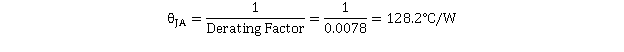Given θJA of 128.2°C/W, the maximum allowable junction temperature of 150°C, and the maximum internal dissipation of 0.4 W (2.25 W, 4-Ω load, 5-V supply, from Figure 3), the maximum ambient temperature can be calculated with the following equation.

Equation 2.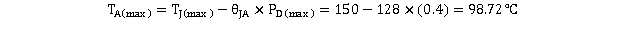Equation 2 shows that the calculated maximum ambient temperature is 98.72°C at maximum power dissipation with a 5-V supply and 4-Ω a load. See Figure 3. The TPA2010D1 is designed with thermal protection that turns the device off when the junction temperature surpasses 165°C ~ 190°C to prevent damage to the IC. Using speakers more resistive than 4-Ω dramatically increases the thermal performance by reducing the output current and increasing the efficiency of the amplifier.

#### 8.3.4 Eliminating the Output Filter With the TPA2010D1

This section describes why the user can eliminate the output filter with the TPA2010D1.

#### 8.3.4.1 Effect on Audio

The class-D amplifier outputs a pulse-width modulated (PWM) square wave, which is the sum of the switching waveform and the amplified input audio signal. The human ear acts as a band-pass filter such that only the frequencies between approximately 20 Hz and 20 kHz are passed. The switching frequency components are much greater than 20 kHz, so the only signal heard is the amplified input audio signal.

#### 8.3.4.2 Traditional Class-D Modulation Scheme

The traditional class-D modulation scheme, which is used in the TPA005Dxx family, has a differential output where each output is 180 degrees out of phase and changes from ground to the supply voltage, VDD. Therefore, the differential pre-filtered output varies between positive and negative VDD, where filtered 50% duty cycle yields 0 volts across the load. The traditional class-D modulation scheme with voltage and current waveforms is shown in Figure 27.

NOTE

Even at an average of 0 volts across the load (50% duty cycle), the current to the load is high causing a high loss and thus causing a high supply current.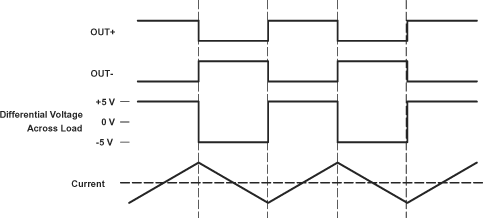Figure 27. Traditional Class-D Modulation Scheme's Output Voltage and Current Waveforms into an Inductive Load with No Input

#### 8.3.4.3 TPA2010D1 Modulation Scheme

The TPA2010D1 uses a modulation scheme that still has each output switching from 0 to the supply voltage. However, OUT+ and OUT– are now in phase with each other with no input. The duty cycle of OUT+ is greater than 50% and OUT– is less than 50% for positive voltages. The duty cycle of OUT+ is less than 50% and OUT– is greater than 50% for negative voltages. The voltage across the load sits at 0 volts throughout most of the switching period greatly reducing the switching current, which reduces any I2R losses in the load.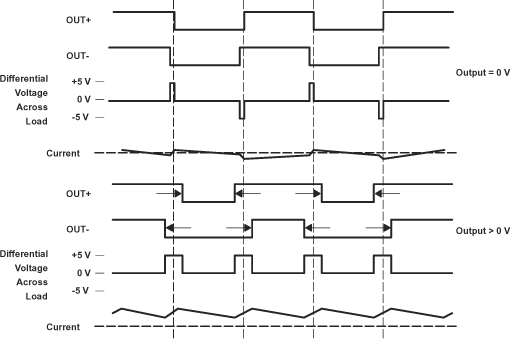Figure 28. The TPA2010D1 Output Voltage and Current Waveforms into an Inductive Load

#### 8.3.4.4 Efficiency: Use a Filter With the Traditional Class-D Modulation Scheme

The main reason that the traditional class-D amplifier needs an output filter is that the switching waveform results in maximum current flow. This causes more loss in the load, causing lower efficiency. The ripple current is large for the traditional modulation scheme because the ripple current is proportional to voltage multiplied by the time at that voltage. The differential voltage swing is 2 × VDD and the time at each voltage is half the period for the traditional modulation scheme. An ideal LC filter is needed to store the ripple current from each half cycle for the next half cycle, while any resistance causes power dissipation. The speaker is both resistive and reactive, whereas an LC filter is almost purely reactive.

The TPA2010D1 modulation scheme has very little loss in the load without a filter because the pulses are very short and the change in voltage is VDD instead of 2 × VDD. As the output power increases, the pulses widen making the ripple current larger. Ripple current can be filtered with an LC filter for increased efficiency, but for most applications the filter is not needed.

An LC filter with a cutoff frequency less than the class-D switching frequency allows the switching current to flow through the filter instead of the load. The filter has less resistance than the speaker that results in less power dissipated, which increases efficiency.

#### 8.3.4.5 Effects of Applying a Square Wave into a Speaker

If the amplitude of a square wave is high enough and the frequency of the square wave is within the bandwidth of the speaker, a square wave causes the voice coil to jump out of the air gap and/or scar the voice coil. However, a 250-kHz switching frequency is not significant because the speaker cone movement is proportional to 1/f2 for frequencies beyond the audio band. Therefore, the amount of cone movement at the switching frequency is very small. However, damage could occur to the speaker if the voice coil is not designed to handle the additional power. To size the speaker for added power, the ripple current dissipated in the load needs to be calculated by subtracting the theoretical supplied power, PSUP THEORETICAL, from the actual supply power, PSUP, at maximum output power, POUT. The switching power dissipated in the speaker is the inverse of the measured efficiency, ηMEASURED, minus the theoretical efficiency, ηTHEORETICAL.

Equation 3.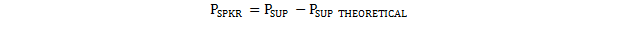where

• the speaker is operating at maximum power
Equation 4.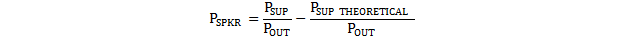Equation 5.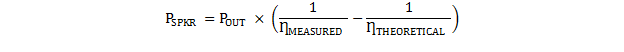Equation 6.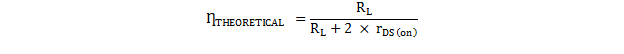The maximum efficiency of the TPA2010D1 with a 3.6 V supply and an 8-Ω load is 86% from Equation 6. Using equation Equation 5 with the efficiency at maximum power (84%), we see that there is an additional 17 mW dissipated in the speaker. The added power dissipated in the speaker is not an issue as long as it is taken into account when choosing the speaker.

#### 8.3.4.6 When to Use an Output Filter

Design the TPA2010D1 without an output filter if the traces from amplifier to speaker are short. The TPA2010D1 passed FCC and CE radiated emissions with no shielding with speaker trace wires 100 mm long or less. Wireless handsets and PDAs are great applications for class-D without a filter.

A ferrite bead filter can often be used if the design is failing radiated emissions without an LC filter, and the frequency sensitive circuit is greater than 1 MHz. This is good for circuits that just have to pass FCC and CE because FCC and CE only test radiated emissions greater than 30 MHz. If choosing a ferrite bead, choose one with high impedance at high frequencies, but very low impedance at low frequencies.

Use an LC output filter if there are low frequency (< 1 MHz) EMI sensitive circuits and/or there are long leads from amplifier to speaker.

Figure 29 and Figure 30 show typical ferrite bead and LC output filters.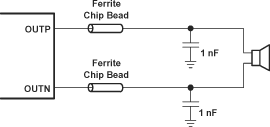Figure 29. Typical Ferrite Chip Bead Filter (Chip Bead Example: NEC/Tokin: N2012zps121)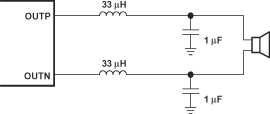Figure 30. Typical LC Output Filter, Cutoff Frequency Of 27 kHz

### 8.4 Device Functional Modes

#### 8.4.1 Summing Input Signals with the TPA2010D1

Most wireless phones or PDAs must sum signals at the audio power amplifier or have two signals sources that need separate gain. The TPA2010D1 makes it easy to sum signals or use separate signal sources with different gains. Many phones now use the same speaker for the ear-piece and ringer, where the wireless phone would require a much lower gain for the phone ear-piece than for the ringer. PDAs and phones that have stereo headphones require summing of the right and left channels to output the stereo signal to the mono speaker.

#### 8.4.1.1 Summing Two Differential Inputs

Two extra resistors are needed for summing differential signals (a total of 5 components). The gain for each input source can be set independently (see Equation 7 and Equation 8, and Figure 31).

Equation 7.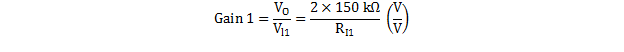Equation 8.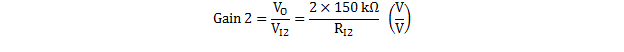If summing left and right inputs with a gain of 1 V/V, use RI1 = RI2 = 300 kΩ.

If summing a ring tone and a phone signal, set the ring-tone gain to Gain 2 = 2 V/V, and the phone gain to gain
1 = 0.1 V/V. The resistor values would be: RI1 = 3 MΩ, and = RI2 = 150 kΩ.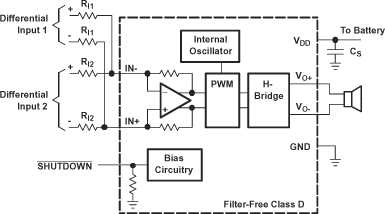Figure 31. TPA2010D1 Summing Two Differential Inputs

#### 8.4.1.2 Summing a Differential Input Signal and a Single-Ended Input Signal

Figure 32 shows how to sum a differential input signal and a single-ended input signal. Ground noise can couple in through IN+ with this method. It is better to use differential inputs. The corner frequency of the single-ended input is set by CI2, shown in Equation 11. To assure that each input is balanced, the single-ended input must be driven by a low-impedance source even if the input is not in use.

Equation 9.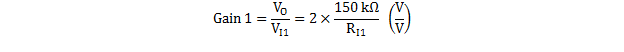Equation 10.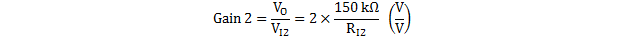Equation 11.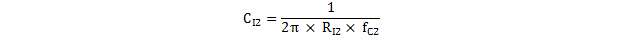If summing a ring tone and a phone signal, the phone signal should use a differential input signal while the ring tone might be limited to a single-ended signal. Phone gain is set at Gain 1 = 0.1 V/V, and the ring-tone gain is set to Gain 2 = 2 V/V, the resistor values would be: RI1 = MΩ, and RI2 = 150 kΩ.

The high pass corner frequency of the single-ended input is set by capacitor CI2. If the desired corner frequency is less than 20 Hz.

Equation 12.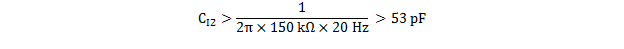Figure 32. TPA2010D1 Summing Differential Input and Single-Ended Input Signals

#### 8.4.1.3 TPA2010D1 Summing Two Single-Ended Inputs

Four resistors and three capacitors are needed for summing single-ended input signals. The gain and corner frequencies (fc1 and fc2) for each input source can be set independently (see Equation 13 through Equation 16, and Figure 33). Resistor, RP, and capacitor, CP, are needed on the IN+ terminal to match the impedance on the IN– terminal. The single-ended inputs must be driven by low impedance sources even if one of the inputs is not outputting an AC signal.

Equation 13.Equation 14.Equation 15.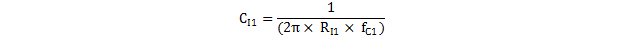Equation 16.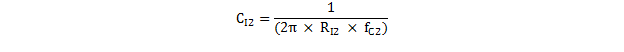Equation 17.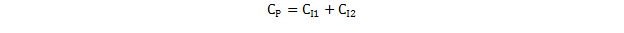Equation 18.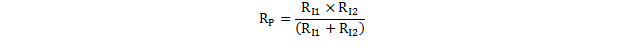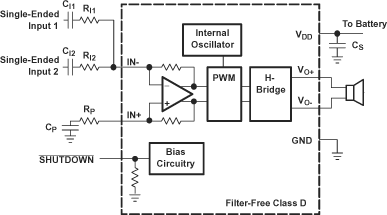Figure 33. TPA2010D1 Summing Two Single-Ended Inputs

#### 8.4.2 Shutdown Mode

The TPA2010D1 can be put in shutdown mode when asserting SHUTDOWN pin to a logic LOW. While in shutdown mode, the device output stage is turned off and set into high impedance, making the current consumption very low. The device exits shutdown mode when a HIGH logic level is applied to the SHUTDOWN pin.